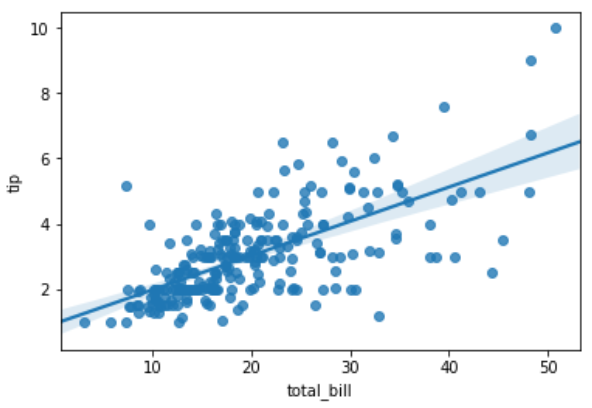# Python – Linear Regression

In Linear Regression these two variables are related through an equation, where exponent (power) of both these variables is 1. Mathematically a linear relationship represents a straight line when plotted as a graph. A non-linear relationship where the exponent of any variable is not equal to 1 creates a curve.

The functions in Seaborn to find the linear regression relationship is regplot. The below example shows its use.

```
import seaborn as sb
from matplotlib import pyplot as plt